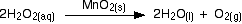# The Effect of Concentration on Rate of Reaction

•• Contributed by Jim Clark
• Former Head of Chemistry and Head of Science at Truro School in Cornwall

This page describes and explains the way that changing the concentration of a solution affects the rate of a reaction. Be aware that this is an introductory page only.

## Introduction

For many reactions involving liquids or gases, increasing the concentration of the reactants increases the rate of reaction. In a few cases, increasing the concentration of one of the reactants may have little noticeable effect of the rate. These cases are discussed and explained further down this page. Don't assume that if you double the concentration of one of the reactants that you will double the rate of the reaction. It may happen like that, but the relationship may well be more complicated.

Example $$\PageIndex{1}$$: Zinc and hydrochloric acid

In the lab, zinc granules react fairly slowly with dilute hydrochloric acid, but much faster if the acid is concentrated.

$Zn(s) + 2HCl (aq) \rightarrow ZnCl_2 (aq) + H_2 (g)$

Example $$\PageIndex{2}$$: The catalytic decomposition of hydrogen peroxide

Solid manganese(IV) oxide is often used as a catalyst in this reaction. Oxygen is given off much faster if the hydrogen peroxide is concentrated than if it is dilute.Example $$\PageIndex{1}$$: The reaction between sodium thiosulphate solution and hydrochloric acid

This is a reaction which is often used to explore the relationship between concentration and rate of reaction in introductory courses. When a dilute acid is added to sodium thiosulphate solution, a pale yellow precipitate of sulphur is formed.

$Na_2S_2O_3 (aq) + 2HCl (aq) \rightarrow 2NaCl(aq) + H_2O(l) + SO_2 (g)$

As the sodium thiosulphate solution is diluted more and more, the precipitate takes longer and longer to form.

## Cases where changing the concentration affects the rate of the reaction

This is the common case, and is easily explained.

## Collisions involving two particles

The same argument applies whether the reaction involves collision between two different particles or two of the same particle. In order for any reaction to happen, those particles must first collide. This is true whether both particles are in solution, or whether one is in solution and the other a solid. If the concentration is higher, the chances of collision are greater.## Reactions involving only one particle

If a reaction only involves a single particle splitting up in some way, then the number of collisions is irrelevant. What matters now is how many of the particles have enough energy to react at any one time. Suppose that at any one time 1 in a million particles have enough energy to equal or exceed the activation energy. If you had 100 million particles, 100 of them would react. If you had 200 million particles in the same volume, 200 of them would now react. The rate of reaction has doubled by doubling the concentration.

## Cases where changing the concentration doesn't affect the rate of the reaction

At first glance this seems very surprising!

### Where a catalyst is already working as fast as it can (Saturated)

Suppose you are using a small amount of a solid catalyst in a reaction, and a high enough concentration of reactant in solution so that the catalyst surface was totally cluttered up with reacting particles. Increasing the concentration of the solution even more can't have any effect because the catalyst is already working at its maximum capacity.

## In certain multi-step reactions

This is the more important effect from an A' level point of view. Suppose you have a reaction which happens in a series of small steps. These steps are likely to have widely different rates - some fast, some slow. For example, suppose two reactants A and B react together in these two stages:The overall rate of the reaction is going to be governed by how fast A splits up to make X and Y. This is described as the rate determining step of the reaction.

• If you increase the concentration of A, you will increase the chances of this step happening for reasons we've looked at above.
• If you increase the concentration of B, that will undoubtedly speed up the second step, but that makes hardly any difference to the overall rate. You can picture the second step as happening so fast already that as soon as any X is formed, it is immediately pounced on by B. That second reaction is already "waiting around" for the first one to happen.

The best specific examples of reactions of this type comes from organic chemistry. These involve the reaction between a tertiary halogenoalkane (alkyl halide) and a number of possible substances - including hydroxide ions. These are examples of nucleophilic substitution using a mechanism known as SN1.